### Home > CC2MN > Chapter 5 Unit 5 > Lesson CC2: 5.3.1 > Problem5-100

5-100.

The following number lines are missing some numbers. Use the information provided to complete the number lines.

1.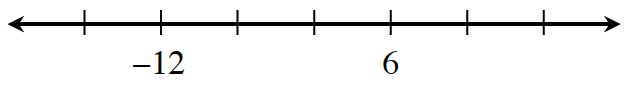Start by finding the difference for one space.

To do this, find the difference between the two given numbers and divide that by the number of spaces between them.

The difference between $-12$ and $6$ is $18$.

$\frac{18}{3}=6.$

The difference for one space is $6$.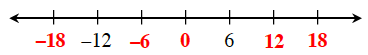The number line has been filled in above for you.

2.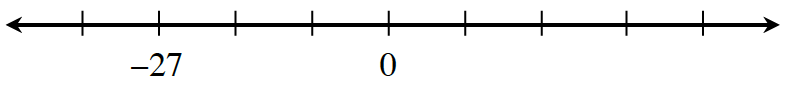Follow the steps in part (a).

The difference between $0$ and $−27$ is $27$.

3.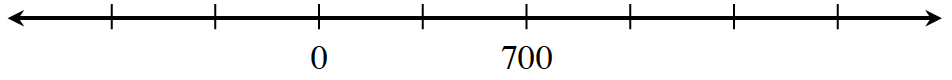Follow the steps in part (a).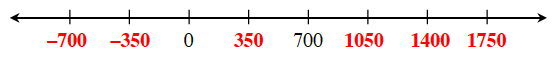4.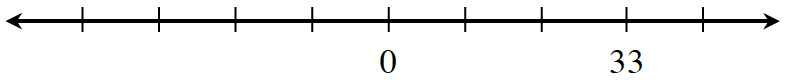Follow the steps in part (a).